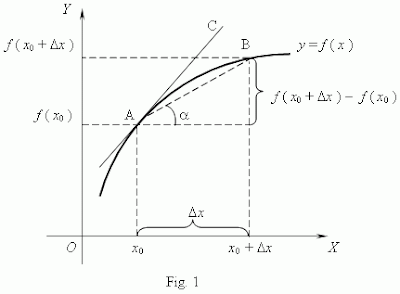## Sunday, October 28, 2007

### The Double Derivative PoemDouble Derivative Poem

The poem above serves as an example of what I am calling a double derivative poem. In the study of mathematics a derivative is the rate of change of one thing as measured by the change in another. The double derivative poem addresses the first and second derivatives of a “phantom function”. The “mathematical function” that I am speaking of is to be experienced with ones intuition as opposed to being explicitly described as a single curve on a Cartesian coordinate system.

The idea for this poetic structure occurred to me while reflecting on the equations from physics that describe the relationship between position velocity and acceleration. Its similar triangles structure dawned on me because physics teaches the position of a moving object is to velocity as velocity is to acceleration.In the study of physics, calculus tells us that the first derivative of position is velocity and the second derivative of position is acceleration. Furthermore, it just so happens that this ‘ratio form’ is the same structure used for a similar triangles poem. (You need to be familiar with the similar triangles poem and mathematical differentiation for this to make sense)
Shown below are visual aids to understand the derivative.The derivative:Animation showing that when delta x approaches zero the secant line becomes tangent. (click on the image to see the animation)Each mathematical term “a” “b” “d” or “e”(see similar triangles poem) is a word or phrase. In the derivative poem each mathematical term is a metaphor relating to the derivative paradigm which is an expression relating the first derivative to the second derivative. (the change and the change in the change)

The first word is mapped into the source domain for the first metaphor in our paradigm serving the target metaphor of ‘position’.

The second word is mapped into the source domain for the second metaphor in our paradigm serving the target metaphor of ‘velocity’.

Caring IS “velocity”

Lastly, the third phrase is mapped into the source domain for the third metaphor in our paradigm serving the target metaphor of ‘acceleration’.
Dailey rituals of love IS “acceleration”

Here is the poem shown in the similar triangles formHere is the poem solved for “Liking”I find the poem more interesting if solved for the word “Caring”Visualizing the exact mathematical function of “Caring as a function of time” would be impossible with the small amount of information given however; a single point on that function is present in the poem even if it is nebulous in form. Furthermore, other poems could be written to describe other points along the phantom curve. I find it very interesting to ponder the shape of the "phantom curve"
The challenge in making a successful derivative poem is in the ability of the poet to express the proper metaphors to fit the paradigm of first and second derivatives. That is to say that the poem expresses a change relative to an idea and then a change in the change relative to that same idea. If all of these requirements are met then one may be able to visualize a point and part of the nebulous function graphically in their mind.

Now to take this idea a little further lets think about this. As I had mentioned before; a derivative is just the rate of change of one thing as measured by the change in another. Furthermore you can have a third derivative, a fourth derivative etc. So in effect we could create a mathematical poem that has its structure as a ‘string or sequence’ of derivatives expressed as an expanded similar triangles poem. Now that sounds like an interesting challenge! Who is going to be the first to do it and send it to me?

also see thisAnonymous said...

When solving for caring, the correct formation would be the square root of (daliy rituals of love*liking). Before solving for the square, the denominator must be taken out correctly.

Kaz Maslanka said...

Yikes!

Making a mistake is embarrassing however, making such a simple one is more so.

Thanks a million!
Kaz